• 运动结构的概念
• 从一对图像估计相机运动
• 重建场景
• 多角度重建
• 优化后的重建
• 可视化 3D 点云

# 运动结构的概念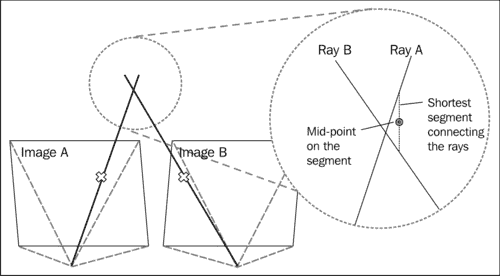# 从一对图像估计相机运动

Mat_<double> E = K.t() * F * K; //according to HZ (9.12)


## 使用丰富特征描述符的点匹配

// detectingkeypoints
SurfFeatureDetectordetector();
vector<KeyPoint> keypoints1, keypoints2;
detector.detect(img1, keypoints1);
detector.detect(img2, keypoints2);

// computing descriptors
SurfDescriptorExtractor extractor;
Mat descriptors1, descriptors2;
extractor.compute(img1, keypoints1, descriptors1);
extractor.compute(img2, keypoints2, descriptors2);

// matching descriptors
BruteForceMatcher<L2<float>> matcher;
vector<DMatch> matches;
matcher.match(descriptors1, descriptors2, matches);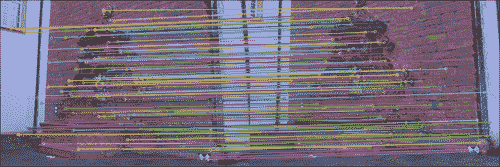## 使用光流的点匹配

### 注意

Vector<KeyPoint>left_keypoints,right_keypoints;

// Detect keypoints in the left and right images
FastFeatureDetectorffd;
ffd.detect(img1, left_keypoints);
ffd.detect(img2, right_keypoints);

vector<Point2f>left_points;
KeyPointsToPoints(left_keypoints,left_points);

vector<Point2f>right_points(left_points.size());

// making sure images are grayscale
Mat prevgray,gray;
if (img1.channels() == 3) {
cvtColor(img1,prevgray,CV_RGB2GRAY);
cvtColor(img2,gray,CV_RGB2GRAY);
} else {
prevgray = img1;
gray = img2;
}

// Calculate the optical flow field:
//  how each left_point moved across the 2 images
vector<uchar>vstatus; vector<float>verror;
calcOpticalFlowPyrLK(prevgray, gray, left_points, right_points, vstatus, verror);

// First, filter out the points with high error
vector<Point2f>right_points_to_find;
vector<int>right_points_to_find_back_index;
for (unsigned inti=0; i<vstatus.size(); i++) {
if (vstatus[i] &&verror[i] < 12.0) {
// Keep the original index of the point in the
// optical flow array, for future use
right_points_to_find_back_index.push_back(i);
// Keep the feature point itself
right_points_to_find.push_back(j_pts[i]);
} else {
vstatus[i] = 0; // a bad flow
}
}

// for each right_point see which detected feature it belongs to
Mat right_points_to_find_flat = Mat(right_points_to_find).reshape(1,to_find.size()); //flatten array

vector<Point2f>right_features; // detected features
KeyPointsToPoints(right_keypoints,right_features);

Mat right_features_flat = Mat(right_features).reshape(1,right_features.size());

// Look around each OF point in the right image
//  for any features that were detected in its area
//  and make a match.
BFMatchermatcher(CV_L2);
vector<vector<DMatch>>nearest_neighbors;
right_points_to_find_flat,
right_features_flat,
nearest_neighbors,
2.0f);

// Check that the found neighbors are unique (throw away neighbors
//  that are too close together, as they may be confusing)
std::set<int>found_in_right_points; // for duplicate prevention
for(inti=0;i<nearest_neighbors.size();i++) {
DMatch _m;
if(nearest_neighbors[i].size()==1) {
_m = nearest_neighbors[i]; // only one neighbor
} else if(nearest_neighbors[i].size()>1) {
// 2 neighbors – check how close they are
double ratio = nearest_neighbors[i].distance / nearest_neighbors[i].distance;
if(ratio < 0.7) { // not too close
// take the closest (first) one
_m = nearest_neighbors[i];
} else { // too close – we cannot tell which is better
continue; // did not pass ratio test – throw away
}
} else {
continue; // no neighbors... :(
}

// prevent duplicates
if (found_in_right_points.find(_m.trainIdx) == found_in_right_points.end()) {
// The found neighbor was not yet used:
// We should match it with the original indexing
// ofthe left point
_m.queryIdx = right_points_to_find_back_index[_m.queryIdx];
found_in_right_points.insert(_m.trainIdx);
}
}
cout<<"pruned "<< matches->size() <<" / "<<nearest_neighbors.size() <<" matches"<<endl;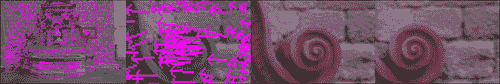## 查找相机矩阵

vector<Point2f>imgpts1,imgpts2;
for( unsigned inti = 0; i<matches.size(); i++ )
{
// queryIdx is the "left" image
imgpts1.push_back(keypoints1[matches[i].queryIdx].pt);
// trainIdx is the "right" image
imgpts2.push_back(keypoints2[matches[i].trainIdx].pt);
}

Mat F = findFundamentalMat(imgpts1, imgpts2, FM_RANSAC, 0.1, 0.99, status);
Mat_<double> E = K.t() * F * K; //according to HZ (9.12)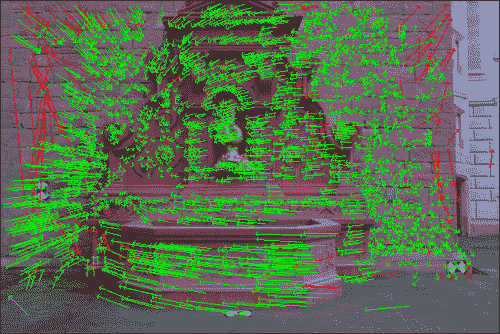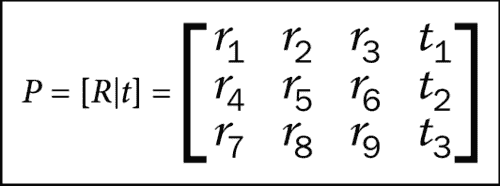SVD svd(E);
Matx33d W(0,-1,0,//HZ 9.13
1,0,0,
0,0,1);
Mat_<double> R = svd.u * Mat(W) * svd.vt; //HZ 9.19
Mat_<double> t = svd.u.col(2); //u3
Matx34d P1(  R(0,0),R(0,1), R(0,2), t(0),
R(1,0),R(1,1), R(1,2), t(1),
R(2,0),R(2,1), R(2,2), t(2));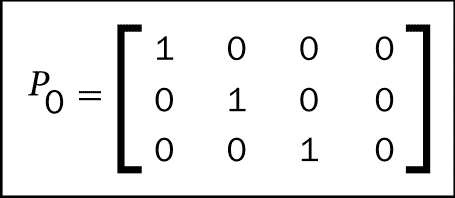bool CheckCoherentRotation(cv::Mat_<double>& R) {
if(fabsf(determinant(R))-1.0 > 1e-07) {
cerr<<"det(R) != +-1.0, this is not a rotation matrix"<<endl;
return false;
}
return true;
}


void FindCameraMatrices(const Mat& K,
const Mat& Kinv,
const vector<KeyPoint>& imgpts1,
const vector<KeyPoint>& imgpts2,
Matx34d& P,
Matx34d& P1,
vector<DMatch>& matches,
vector<CloudPoint>& outCloud
)
{
//Find camera matrices

//Get Fundamental Matrix
Mat F = GetFundamentalMat(imgpts1,imgpts2,matches);

//Essential matrix: compute then extract cameras [R|t]
Mat_<double> E = K.t() * F * K; //according to HZ (9.12)

//decompose E to P' , HZ (9.19)
SVD svd(E,SVD::MODIFY_A);
Mat svd_u = svd.u;
Mat svd_vt = svd.vt;
Mat svd_w = svd.w;

Matx33d W(0,-1,0,//HZ 9.13
1,0,0,
0,0,1);
Mat_<double> R = svd_u * Mat(W) * svd_vt; //HZ 9.19
Mat_<double> t = svd_u.col(2); //u3

if (!CheckCoherentRotation(R)) {
cout<<"resulting rotation is not coherent\n";
P1 = 0;
return;
}

P1 = Matx34d(R(0,0),R(0,1),R(0,2),t(0),
R(1,0),R(1,1),R(1,2),t(1),
R(2,0),R(2,1),R(2,2),t(2));
}


# 重建场景

Mat_<double> LinearLSTriangulation(
Point3d u,//homogenous image point (u,v,1)
Matx34d P,//camera 1 matrix
Point3d u1,//homogenous image point in 2nd camera
Matx34d P1//camera 2 matrix
)
{
//build A matrix
Matx43d A(u.x*P(2,0)-P(0,0),u.x*P(2,1)-P(0,1),u.x*P(2,2)-P(0,2),
u.y*P(2,0)-P(1,0),u.y*P(2,1)-P(1,1),u.y*P(2,2)-P(1,2),
u1.x*P1(2,0)-P1(0,0), u1.x*P1(2,1)-P1(0,1),u1.x*P1(2,2)-P1(0,2),
u1.y*P1(2,0)-P1(1,0), u1.y*P1(2,1)-P1(1,1),u1.y*P1(2,2)-P1(1,2)
);
//build B vector
Matx41d B(-(u.x*P(2,3)-P(0,3)),
-(u.y*P(2,3)-P(1,3)),
-(u1.x*P1(2,3)-P1(0,3)),
-(u1.y*P1(2,3)-P1(1,3)));

//solve for X
Mat_<double> X;
solve(A,B,X,DECOMP_SVD);

return X;
}


double TriangulatePoints(
const vector<KeyPoint>& pt_set1,
const vector<KeyPoint>& pt_set2,
const Mat&Kinv,
const Matx34d& P,
const Matx34d& P1,
vector<Point3d>& pointcloud)
{
vector<double> reproj_error;
for (unsigned int i=0; i<pts_size; i++) {
//convert to normalized homogeneous coordinates
Point2f kp = pt_set1[i].pt;
Point3d u(kp.x,kp.y,1.0);
Mat_<double> um = Kinv * Mat_<double>(u);
u = um.at<Point3d>(0);
Point2f kp1 = pt_set2[i].pt;
Point3d u1(kp1.x,kp1.y,1.0);
Mat_<double> um1 = Kinv * Mat_<double>(u1);
u1 = um1.at<Point3d>(0);

//triangulate
Mat_<double> X = LinearLSTriangulation(u,P,u1,P1);

//calculate reprojection error
Mat_<double> xPt_img = K * Mat(P1) * X;
Point2f xPt_img_(xPt_img(0)/xPt_img(2),xPt_img(1)/xPt_img(2));
reproj_error.push_back(norm(xPt_img_-kp1));

//store 3D point
pointcloud.push_back(Point3d(X(0),X(1),X(2)));
}

//return mean reprojection error
Scalar me = mean(reproj_error);
return me;
}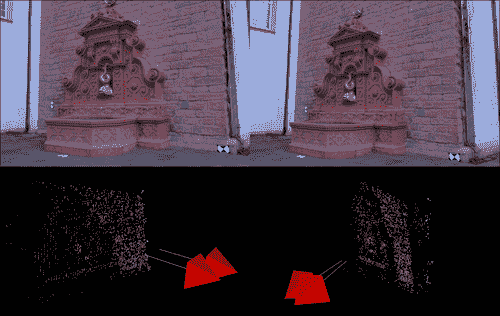# 多角度重建

struct CloudPoint {
cv::Point3d pt;
std::vector<int>index_of_2d_origin;
};


std::vector<CloudPoint> pcloud; //our global 3D point cloud

//check for matches between i'th frame and 0'th frame (and thus the current cloud)
std::vector<cv::Point3f> ppcloud;
std::vector<cv::Point2f> imgPoints;
vector<int> pcloud_status(pcloud.size(),0);

//scan the views we already used (good_views)
for (set<int>::iterator done_view = good_views.begin(); done_view != good_views.end(); ++done_view)
{
int old_view = *done_view; //a view we already used for reconstrcution
//check for matches_from_old_to_working between <working_view>'th frame and <old_view>'th frame (and thus the current cloud)
std::vector<cv::DMatch> matches_from_old_to_working = matches_matrix[std::make_pair(old_view,working_view)];
//scan the 2D-2D matched-points
for (unsigned int match_from_old_view=0; match_from_old_view<matches_from_old_to_working.size(); match_from_old_view++) {
// the index of the matching 2D point in <old_view>
int idx_in_old_view = matches_from_old_to_working[match_from_old_view].queryIdx;

//scan the existing cloud to see if this point from <old_view> exists for (unsigned int pcldp=0; pcldp<pcloud.size(); pcldp++) {
// see if this 2D point from <old_view> contributed to this 3D point in the cloud
if (idx_in_old_view == pcloud[pcldp].index_of_2d_origin[old_view] && pcloud_status[pcldp] == 0) //prevent duplicates
{
//3d point in cloud
ppcloud.push_back(pcloud[pcldp].pt);
//2d point in image <working_view>
Point2d pt_ = imgpts[working_view][matches_from_old_to_working[match_from_old_view].trainIdx].pt;
imgPoints.push_back(pt_);

pcloud_status[pcldp] = 1;
break;
}
}
}
}
cout<<"found "<<ppcloud.size() <<" 3d-2d point correspondences"<<endl;


cv::Mat_<double> t,rvec,R;
cv::solvePnPRansac(ppcloud, imgPoints, K, distcoeff, rvec, t, false);

//get rotation in 3x3 matrix form
Rodrigues(rvec, R);

P1 = cv::Matx34d(R(0,0),R(0,1),R(0,2),t(0),
R(1,0),R(1,1),R(1,2),t(1),
R(2,0),R(2,1),R(2,2),t(2));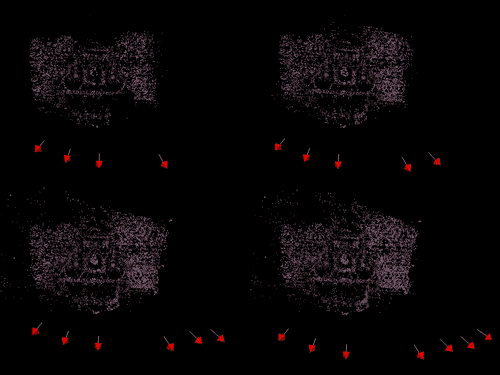# 优化后的重建

SfM 方法最重要的部分之一是优化和优化重建的场景，也称为包调整BA）的过程。 此是优化步骤，其中，我们收集的所有数据均拟合为整体模型。 3D 点的位置和相机的位置都得到了优化，因此重投影误差最小化（即，将近似的 3D 点投影到图像上接近原始 2D 点的位置）。 该过程通常需要求解成千上万个参数的非常大的线性方程。 该过程可能会有些费力，但是我们之前采取的步骤将使与 Bundle Adjuster 的集成变得容易。 以前看起来有些奇怪的某些事情可能会变得清晰起来。 例如，我们为云中的每个 3D 点保留原点 2D 点的原因。

voidBundleAdjuster::adjustBundle(
vector<CloudPoint>&pointcloud,
const Mat&cam_intrinsics,
conststd::vector<std::vector<cv::KeyPoint>>&imgpts,
std::map<int ,cv::Matx34d>&Pmats
)
{
int N = Pmats.size(), M = pointcloud.size(), K = -1;

cout<<"N (cams) = "<< N <<" M (points) = "<< M <<" K (measurements) = "<< K <<endl;

StdDistortionFunction distortion;

// intrinsic parameters matrix
Matrix3x3d KMat;
makeIdentityMatrix(KMat);
KMat = cam_intrinsics.at<double>(0,0);
KMat = cam_intrinsics.at<double>(0,1);
KMat = cam_intrinsics.at<double>(0,2);
KMat = cam_intrinsics.at<double>(1,1);
KMat = cam_intrinsics.at<double>(1,2);

...

// 3D point cloud
vector<Vector3d >Xs(M);
for (int j = 0; j < M; ++j)
{
Xs[j] = pointcloud[j].pt.x;
Xs[j] = pointcloud[j].pt.y;
Xs[j] = pointcloud[j].pt.z;
}

// convert cameras to BA datastructs
vector<CameraMatrix> cams(N);
for (inti = 0; i< N; ++i)
{
intcamId = i;
Matrix3x3d R;
Vector3d T;

Matx34d& P = Pmats[i];

R = P(0,0); R = P(0,1); R = P(0,2); T = P(0,3);
R = P(1,0); R = P(1,1); R = P(1,2); T = P(1,3);
R = P(2,0); R = P(2,1); R = P(2,2); T = P(2,3);

cams[i].setIntrinsic(Knorm);
cams[i].setRotation(R);
cams[i].setTranslation(T);
}

vector<Vector2d > measurements;
vector<int> correspondingView;
vector<int> correspondingPoint;

// 2D corresponding points
for (unsigned int k = 0; k <pointcloud.size(); ++k)
{
for (unsigned int i=0; i<pointcloud[k].imgpt_for_img.size(); i++) {
if (pointcloud[k].imgpt_for_img[i] >= 0) {
int view = i, point = k;
Vector3d p, np;

Point cvp = imgpts[i][pointcloud[k].imgpt_for_img[i]].pt;
p = cvp.x;
p = cvp.y;
p = 1.0;

// Normalize the measurements to match the unit focal length.
scaleVectorIP(1.0/f0, p);
measurements.push_back(Vector2d(p, p));
correspondingView.push_back(view);
correspondingPoint.push_back(point);
}
}
} // end for (k)

K = measurements.size();

cout<<"Read "<< K <<" valid 2D measurements."<<endl;

...

{
CommonInternalsMetricBundleOptimizeropt(V3D::FULL_BUNDLE_FOCAL_LENGTH_PP, inlierThreshold, K0, distortion, cams, Xs, measurements, correspondingView, correspondingPoint);

opt.tau = 1e-3;
opt.maxIterations = 50;
opt.minimize();

cout<<"optimizer status = "<<opt.status<<endl;
}

...

//extract 3D points
for (unsigned int j = 0; j <Xs.size(); ++j)
{
pointcloud[j].pt.x = Xs[j];
pointcloud[j].pt.y = Xs[j];
pointcloud[j].pt.z = Xs[j];
}
for (int i = 0; i< N; ++i)
{
Matrix3x3d R = cams[i].getRotation();
Vector3d T = cams[i].getTranslation();
Matx34d P;
P(0,0) = R; P(0,1) = R; P(0,2) = R; P(0,3) = T;
P(1,0) = R; P(1,1) = R; P(1,2) = R; P(1,3) = T;
P(2,0) = R; P(2,1) = R; P(2,2) = R; P(2,3) = T;
Pmats[i] = P;
}
}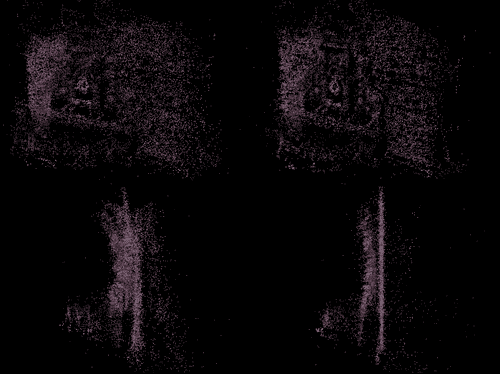# 使用 PCL 可视化 3D 点云

pcl::PointCloud<pcl::PointXYZRGB>::Ptr cloud;

void PopulatePCLPointCloud(const vector<Point3d>& pointcloud,
const std::vector<cv::Vec3b>& pointcloud_RGB
)
//Populate point cloud
{
cout<<"Creating point cloud...";
cloud.reset(new pcl::PointCloud<pcl::PointXYZRGB>);

for (unsigned int i=0; i<pointcloud.size(); i++) {
// get the RGB color value for the point
Vec3b rgbv(255,255,255);
if (pointcloud_RGB.size() >= i) {
rgbv = pointcloud_RGB[i];
}

// check for erroneous coordinates (NaN, Inf, etc.)
if (pointcloud[i].x != pointcloud[i].x || isnan(pointcloud[i].x) ||
pointcloud[i].y != pointcloud[i].y || isnan(pointcloud[i].y) ||
pointcloud[i].z != pointcloud[i].z || isnan(pointcloud[i].z) ||
fabsf(pointcloud[i].x) > 10.0 ||
fabsf(pointcloud[i].y) > 10.0 ||
fabsf(pointcloud[i].z) > 10.0) {
continue;
}

pcl::PointXYZRGB pclp;

// 3D coordinates
pclp.x = pointcloud[i].x;
pclp.y = pointcloud[i].y;
pclp.z = pointcloud[i].z;

// RGB color, needs to be represented as an integer
uint32_t rgb = ((uint32_t)rgbv << 16 | (uint32_t)rgbv << 8 | (uint32_t)rgbv);
pclp.rgb = *reinterpret_cast<float*>(&rgb);

cloud->push_back(pclp);
}

cloud->width = (uint32_t) cloud->points.size(); // number of points
cloud->height = 1; // a list of points, one row of data
}


Void SORFilter() {

pcl::PointCloud<pcl::PointXYZRGB>::Ptr cloud_filtered (new pcl::PointCloud<pcl::PointXYZRGB>);

std::cerr<<"Cloud before SOR filtering: "<< cloud->width * cloud->height <<" data points"<<std::endl;

// Create the filtering object
pcl::StatisticalOutlierRemoval<pcl::PointXYZRGB>sor;
sor.setInputCloud (cloud);
sor.setMeanK (50);
sor.setStddevMulThresh (1.0);
sor.filter (*cloud_filtered);

std::cerr<<"Cloud after SOR filtering: "<<cloud_filtered->width * cloud_filtered->height <<" data points "<<std::endl;

copyPointCloud(*cloud_filtered,*cloud);
}


Void RunVisualization(const vector<cv::Point3d>& pointcloud,
const std::vector<cv::Vec3b>& pointcloud_RGB) {
PopulatePCLPointCloud(pointcloud,pointcloud_RGB);
SORFilter();
copyPointCloud(*cloud,*orig_cloud);

pcl::visualization::CloudViewer viewer("Cloud Viewer");

// run the cloud viewer
viewer.showCloud(orig_cloud,"orig");

while (!viewer.wasStopped ())
{
// NOP
}
}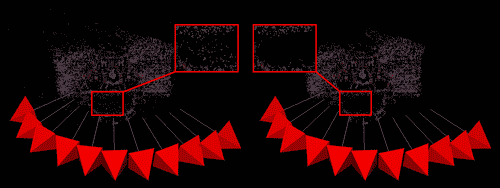# 使用示例代码

• OpenCV v2.3 或更高版本
• PCL v1.6 或更高版本
• SSBA v3.0 或更高版本

cmake –G "Visual Studio 10" -DSSBA_LIBRARY_DIR=../3rdparty/SSBA-3.0/build/ ..



cmake –G "Unix Makefiles" -DSSBA_LIBRARY_DIR=../3rdparty/SSBA-3.0/build/ ..



cmake –G Xcode -DSSBA_LIBRARY_DIR=../3rdparty/SSBA-3.0/build/ ..



CMake 还具有为 Eclipse，代码块等构建宏的能力。 完成 CMake 的创建环境后，我们就可以开始构建了。 如果我们使用的是类似 Unix 的系统，我们可以简单地执行 make 工具，否则我们应该使用开发环境的构建过程。